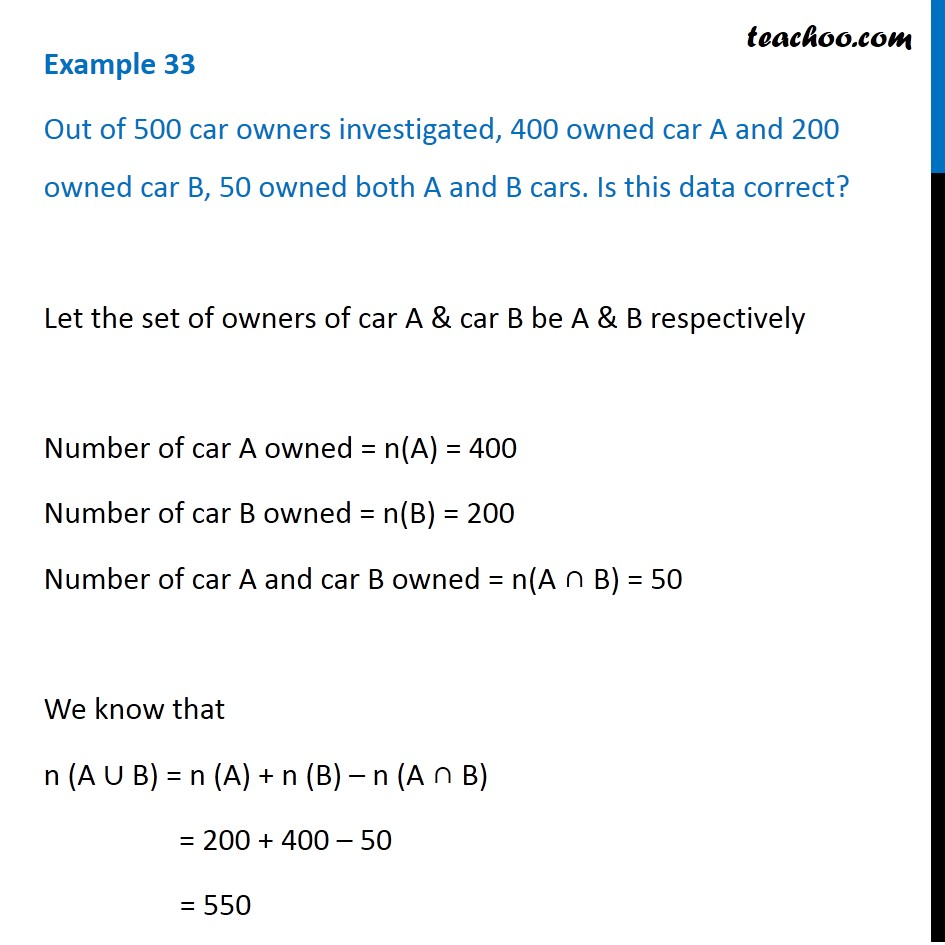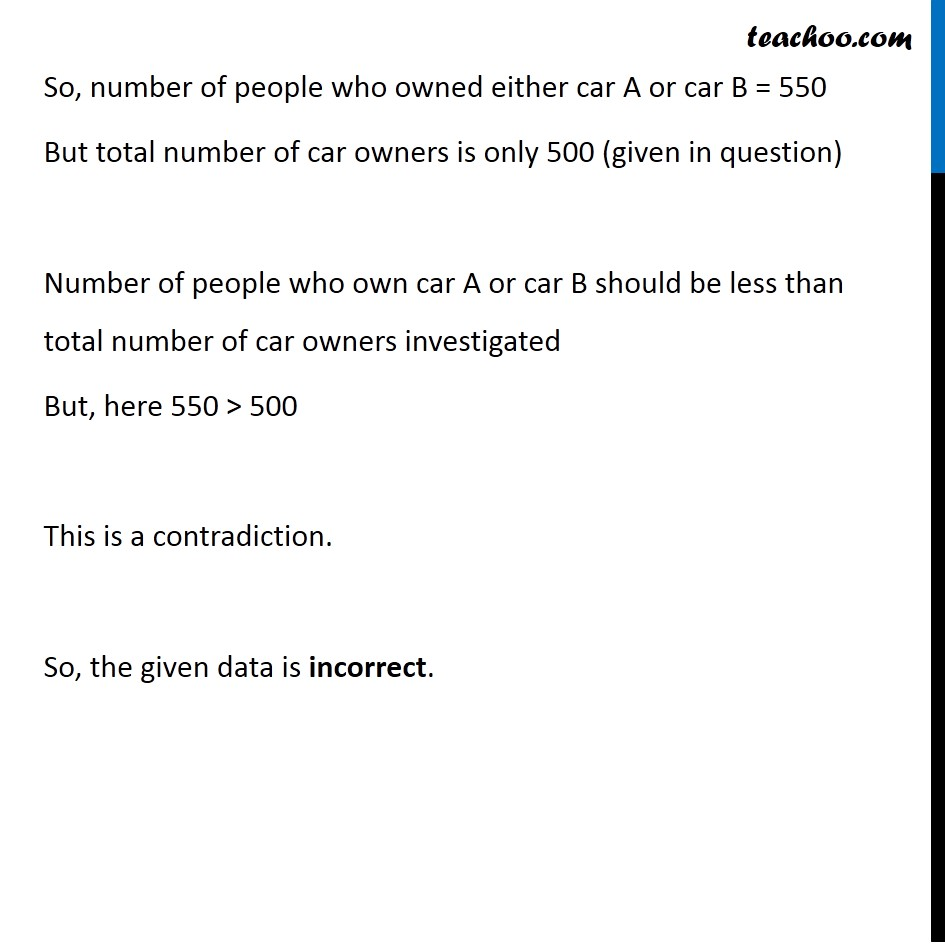Examples

Chapter 1 Class 11 Sets
Serial order wiseLearn in your speed, with individual attention - Teachoo Maths 1-on-1 Class

### Transcript

Question 8 Out of 500 car owners investigated, 400 owned car A and 200 owned car B, 50 owned both A and B cars. Is this data correct? Let the set of owners of car A & car B be A & B respectively Number of car A owned = n(A) = 400 Number of car B owned = n(B) = 200 Number of car A and car B owned = n(A ∩ B) = 50 We know that n (A ∪ B) = n (A) + n (B) – n (A ∩ B) = 200 + 400 – 50 = 550 So, number of people who owned either car A or car B = 550 But total number of car owners is only 500 (given in question) Number of people who own car A or car B should be less than total number of car owners investigated But, here 550 > 500 This is a contradiction. So, the given data is incorrect.